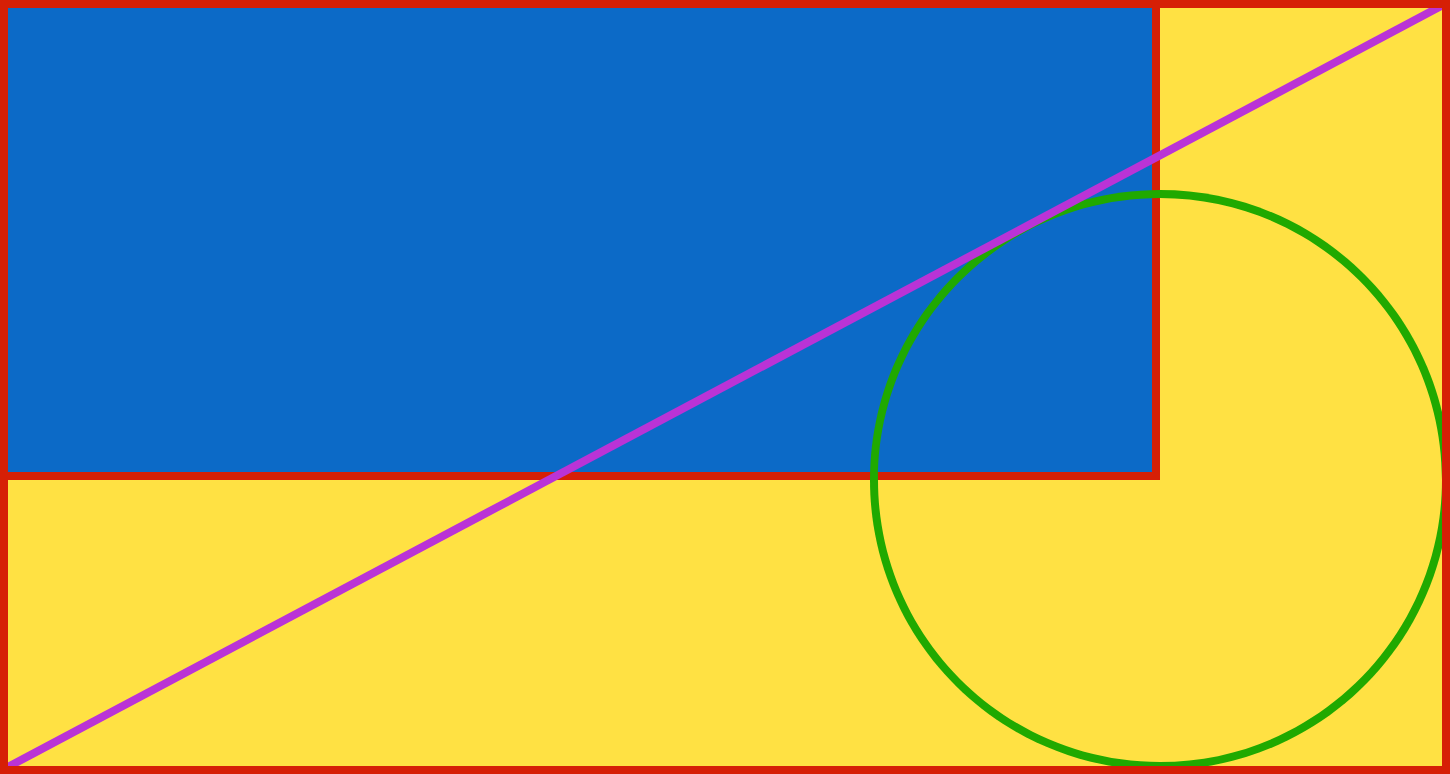# Circle in a rectangle

Geometry Level 1

The large rectangle below is divided into two congruent triangles by one of its diagonals. We inscribe a circle into one of the triangles, and then drop perpendiculars from the center of the circle to two sides of the large rectangle, as shown in the diagram.Which has a larger area, the resulting blue rectangle or the remaining yellow hexagon?

×